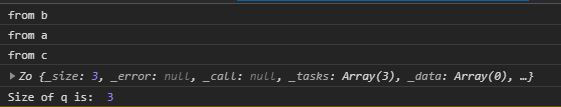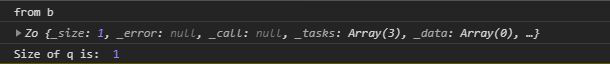Related Articles

# D3.js queue.defer() Function

• Last Updated : 04 Aug, 2020

The queue.defer() function in d3.js is used to add the asynchronous task callback to the queue. Where the task is a function to be executed. The callback must be executed when the task got finished.

Syntax:

```queue.defer(task[, arguments…]);
```

Parameters: This function accepts a single parameter as mentioned above and described below:

• task: It is a function that is to be executed to perform a particular task.

Return Value: This function returns the object.

Below given are a few examples of the above function.

Example 1: When the size of the queue is equal to the number of queue.defer() calls.

## HTML

 ` ``<``html` `lang``=``"en"``> ``<``head``> ``    ``<``meta` `charset``=``"UTF-8"``> ``    ``<``meta` `name``=``"viewport"``            ``path1tent="``width``=``device``-width, ``                       ``initial-scale``=``1``.0"> ``    ``<``title``>Document `` ``<``style``>`` ``<``body``> ``  ``<``script` `src` `= ``"https://d3js.org/d3.v4.min.js"``> ``  `` ``  ``<``script``>``    ``function a(){``      ``console.log("from a")``    ``}``    ``function b(){``      ``console.log("from b")``    ``}``    ``function c(){``      ``console.log("from c")``    ``}``    ``let q=d3.queue(3)``    ``// Calling defer three times``    ``q.defer(b)``    ``q.defer(a)``    ``q.defer(c)``    ``console.log(q)``    ``console.log("Size of q is: ",q._size)``  `` `` ```

Output:Example 2: When the size of the queue is less than the queue.defer() calls.

## HTML

 ` ``<``html` `lang``=``"en"``> ``<``head``> ``    ``<``meta` `charset``=``"UTF-8"``> ``    ``<``meta` `name``=``"viewport"``            ``path1tent="``width``=``device``-width, ``                       ``initial-scale``=``1``.0"> ``    ``<``title``>Document `` ``<``style``> `` ``<``body``> ``  ``<``script` `src` `= ``"https://d3js.org/d3.v4.min.js"``> ``  `` ``  ``<``script``>``    ``function a(){``      ``console.log("from a")``    ``}``    ``function b(){``      ``console.log("from b")``    ``}``    ``function c(){``      ``console.log("from c")``    ``}``    ``let q=d3.queue(1)``    ``// Calling defer three times but it will add only function``    ``// call b because the size of the queue is one.``    ``q.defer(b)``    ``q.defer(a)``    ``q.defer(c)``    ``console.log(q)``    ``console.log("Size of q is: ",q._size)``  `` `` ```

Output:Hey geek! The constant emerging technologies in the world of web development always keeps the excitement for this subject through the roof. But before you tackle the big projects, we suggest you start by learning the basics. Kickstart your web development journey by learning JS concepts with our JavaScript Course. Now at it’s lowest price ever!

My Personal Notes arrow_drop_up